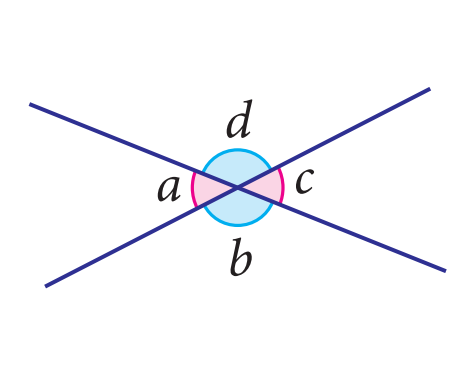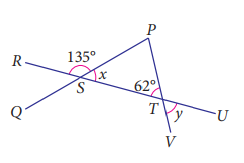## Angles related to Intersecting Lines

 8.2 Angles related to Intersecting Lines

 Example(i) Vertically opposite angles at intersecting lines are equal. \begin{aligned}∠a &= ∠c\\\\ ∠b &= ∠d \end{aligned} (ii) The sum of adjacent angles at intersecting lines is $$180^{\circ}$$. \begin{aligned}∠a+\angle d &= 180^\circ\\\\ ∠d+\angle c&= 180^\circ\\\\ ∠c+\angle b&= 180^\circ\\\\ ∠b+\angle a&= 180^\circ \end{aligned} (iii) If two intersecting lines are perpendicular to each other, then all angles at the intersecting lines have the same size of $$90^{\circ}$$.

Determine the values of the angles at intersecting lines:

 Example The following diagram shows $$PSQ$$, $$RSTU$$ and $$PTV$$ are straight lines.Calculate the values of $$x$$ and $$y$$. Noted that the sum of adjacent angles at intersecting lines is $$180^{\circ}$$. So, \begin{aligned}x + 135^{\circ} &= 180^{\circ} \\\\x&=180^\circ-135^\circ \\\\x&=55^\circ. \end{aligned} Also, the vertically opposite angles at intersecting lines are equal. Thus, $$y = 62^{\circ}$$.